Function Repository Resource:

# TrigContract

Attempt to express weighted sums of sines and cosines having the same period in terms of cosines alone

Contributed by: Wolfram Staff
 ResourceFunction["TrigContract"][expr] evaluates TrigReduce[expr] and attempts to contract weighted sums of sines and cosines with the same period. ResourceFunction["TrigContract"][expr,var] treats all variables other than var as constants.

## Details and Options

ResourceFunction["TrigContract"] attempts to combine terms of the form aSin[x]+bCos[x] into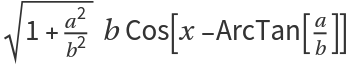.
ResourceFunction["TrigContract"] automatically threads over lists in expr.
Given the option "ExpandTrigsOfSum" True, ResourceFunction["TrigContract"] expands the terms in the form of Sin[α+β] and Cos[α+β] into sines and cosines of α and β.
The default "ExpandTrigsOfSum"Automatic employs heuristics to decide whether to expand the sums.

## Examples

### Basic Examples (1)

Combine sums of sines and cosines:

 In:=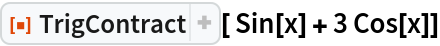Out=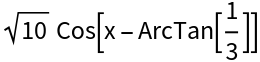In:=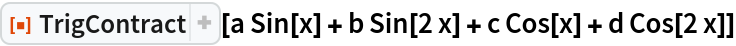Out=### Scope (4)

TrigContract can handle symbolic parameters:

 In:=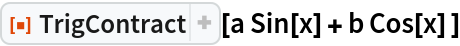Out=Treat x as the main variable and y as a constant:

 In:=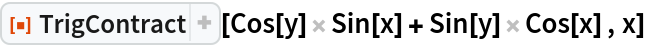Out=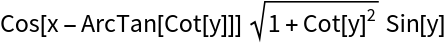Treat y as the main variable and x as a constant:

 In:=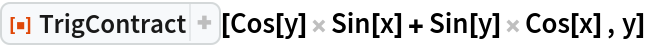Out=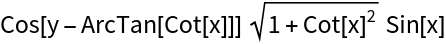Here TrigContract picks y as the main variable and treats x as a constant:

 In:=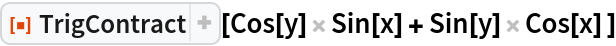Out=In:=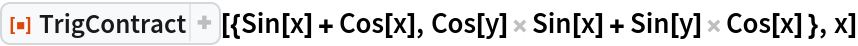Out=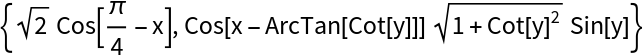Use symbolic manipulations to handle trigonometric functions other than sines and cosines:

 In:=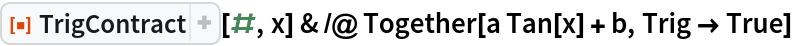Out=### Options (2)

#### ExpandTrigsOfSum (2)

With "ExpandTrigsOfSum"True, TrigContract finds possible contractions for sines and cosines of sums, but may produce relatively more complicated results:

 In:=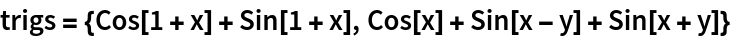Out=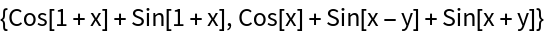In:=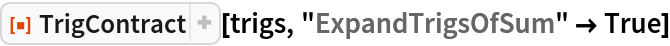Out=Prevent expanding terms in the form (Sin|Cos)[x±y]:

 In:=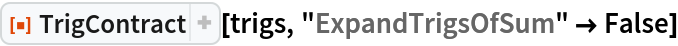Out=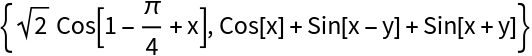### Properties and Relations (2)

TrigContract and TrigExpand are, generically, inverses of each other:

 In:=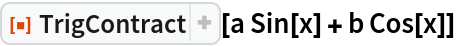Out=In:=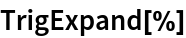Out=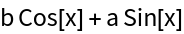TrigContract produces a result equivalent to the result of TrigReduce:

 In:=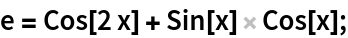In:=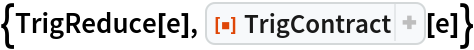Out=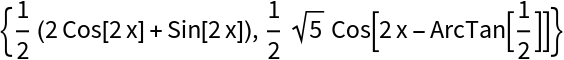In:=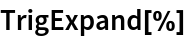Out=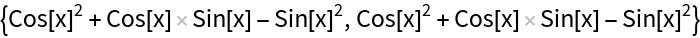### Possible Issues (3)

Without specifying the variable, TrigContract may not always fully contract expr that contains symbolic parameters:

 In:=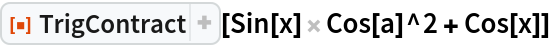Out=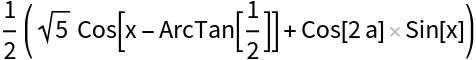Specify the variable:

 In:=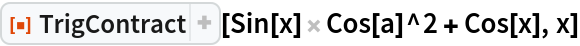Out=Simplify the terms of the product:

 In:=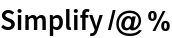Out=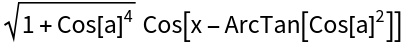## Version History

• 1.0.0 – 02 December 2020